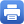Columbia Home
 Home » Programs » Undergraduate Program » Calculus Classes » College Algebra and Analytic Geometry (pre-calculus)College Algebra and Analytic Geometry (pre-calculus) Prerequisites:  A score of 550 on the mathematics portion of the SAT completed within the last year, or the appropriate grade on the General Studies Mathematics Placement Examination. Target Audience: This course is for students who wish to study calculus but do not know analytic geometry. Description: Algebra review, graphs and functions, polynomial functions, rational functions, conic sections, systems of equations in two variables, exponential and logarithmic functions, trigonometric functions and trigonometric identities, applications of trigonometry, sequences, series, and limits. Textbook: James Stewart, Lothar Redlin, Saleem Watson Precalculus: Mathematics for Calculus, 7th edition. The textbook is available at the bookstore, and directly from the publisher. Some sections may use WebAssign. Course Topics: The course covers most of the material in the first eight chapters in the textbook. Properties of real numbers, exponents, linear and quadratic equations, coordinates (Chapter 1) Functions, graphs and their properties, transforming functions (Chapter 2) Polynomials, graphing polynomials, polynomial division, root finding, complex numbers, rational functions (Chapter 3) Exponentials and logarithms (Chapter 4) Trigonometry (Chapters 5 – 7) Polar coordinates, as time permits (Chapter 8) Typical Syllabus: A sample syllabus is available. Calculus Director: Prof. George Dragomir (dragomir@math.columbia.edu) Barnard Calculus Director: Prof. Dusa McDuff (dusa@math.columbia.edu) Help Room Schedule: 502 Milstein Center (Barnard Campus)Print this page